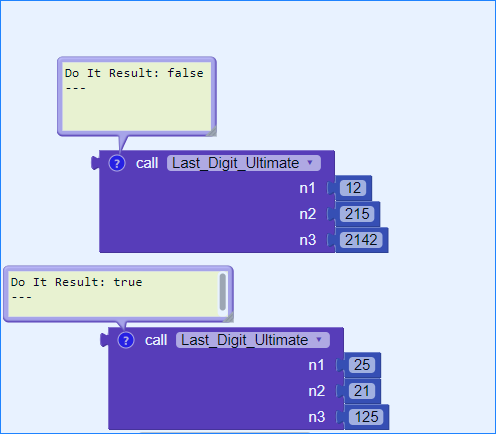# Daily Challenge #40

## Last Digit Ultimate

Your job is to create a procedure, that takes 3 numbers: `a, b, c` and returns `True` if the last digit of (the last digit of `a` * the last digit of `b` ) = the last digit of `c` . Check examples for explanation.

### Examples

``````last_dig(25, 21, 125) ➞ True
# The last digit of 25 is 5, the last digit of 21 is 1, and the last
# digit of 125 is 5, and the last digit of 5*1 = 5, which is equal
# to the last digit of 125(5).

last_dig(55, 226, 5190) ➞ True
# The last digit of 55 is 5, the last digit of 226 is 6, and the last
# digit of 5190 is 0, and the last digit of 5*6 = 30 is 0, which is
# equal to the last digit of 5190(0).

last_dig(12, 215, 2142) ➞ False
# The last digit of 12 is 2, the last digit of 215 is 5, and the last
# digit of 2142 is 2, and the last digit of 2*5 = 10 is 0, which is
# not equal to the last digit of 2142(2).
``````

### Notes

• If you still don’t understand:
• The last digit of a = aa, the last digit of b = bb, and the last digit of c = cc.
• Return `True` if the last digit of aa*bb is equal to cc, and `False` otherwise.
• Numbers can be negative.
3 Likes

Although it was not my day…But I am here with a working(as test shows) solution:-

## Results6 Likes